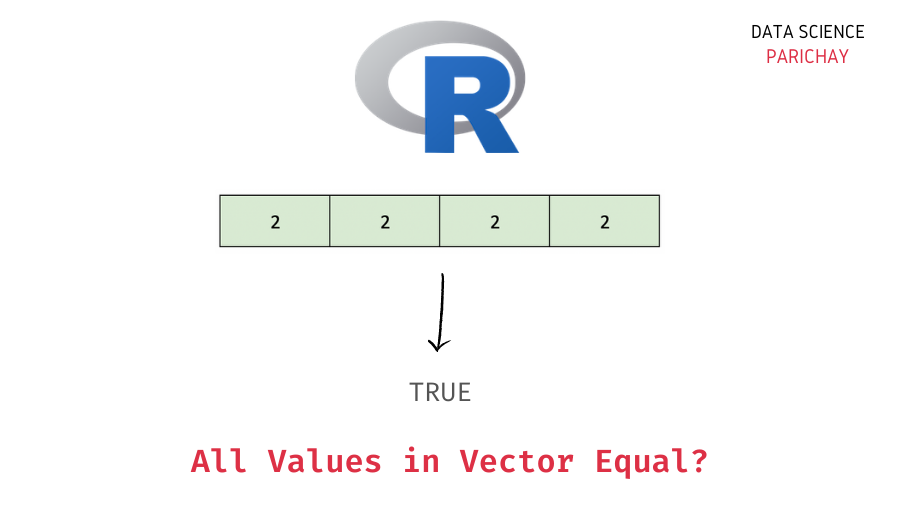# R – Check If All Elements in a Vector are Equal

In this tutorial, we will look at how to check if all the elements in an R vector are equal with the help of some examples.

## How to check if all vector elements are equal in R?

You can use a combination of the `length()` and the `unique()` functions in R to check if all the elements in a vector are equal or not. The following is the syntax –

```# check if all elements in vector are equal
length(unique(vec)) == 1```

Here, we first apply the `unique()` function to keep only the distinct values from the vector and then use the `length()` function to compute the length of this unique values vector.

If the length is equal to 1, we can say that all the elements in the vector are equal. If it were not the case, then the length of the unique value vector would be greater than 1.

## Examples

Let’s now look at some examples of checking for equality among all elements in a vector.

### Using `length()` and `unique()` to check check if all vector elements are equal

Let’s create a vector of length four having the same element in all the indices and apply the method from above.

```# create a vector
vec <- c(7, 7, 7, 7)
# check if all elements in vec are equal
print(length(unique(vec))==1)```

Output:

` TRUE`

We get `TRUE` as the output. This means that the unique values vector has length 1 which indicates that all the values in the vector `vec` are equal.

📚 Data Science Programs By Skill Level

Introductory

Intermediate ⭐⭐⭐

🔎 Find Data Science Programs 👨‍💻 111,889 already enrolled

Disclaimer: Data Science Parichay is reader supported. When you purchase a course through a link on this site, we may earn a small commission at no additional cost to you. Earned commissions help support this website and its team of writers.

### Using `var()` to check if all vector elements are equal

For numeric vectors, you can also use the `var()` function in R to check if all the values are equal or not.

The `var()` function returns the variance in the vector. The idea is, that if the variance of a vector is 0 then all the elements inside the vector are the same.

Let’s look at an example. We’ll use the same input vector as above.

```# create a vector
vec <- c(7, 7, 7, 7)
# check if all elements in vec are equal
print(var(vec)==0)```

Output:

` TRUE`

We get `TRUE` as the output indicating that all the values in the vector are equal.

Note that this method will not work if your vector has non-numeric values. For example, let’s test it on a character vector.

```# create a vector
vec <- c('a', 'a', 'a', 'a')
# check if all elements in vec are equal
print(var(vec)==0)```

Output:

```Warning message in var(vec):
“NAs introduced by coercion”
 NA```

We get `NA` as the output and a warning. This is because we’re trying to compute the variance of a vector with non-numeric values.

Using a combination of the `length()` and the `unique()` function, however, will work irrespective of the type of values the vector has.

```# create a vector
vec <- c('a', 'a', 'a', 'a')
# check if all elements in vec are equal
print(length(unique(vec))==0)```

Output:

` TRUE`

We get `TRUE` as the output.

You might also be interested in –

••